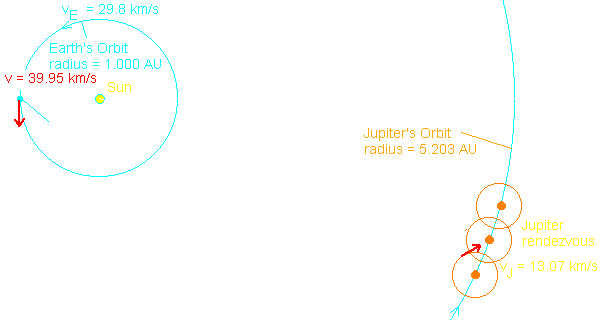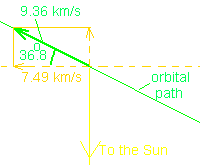### The Orbit of a Space Probe from Earth to Jupiter

#### (In preparation for a gravity assist pass of Jupiter)

Larry Bogan
The diagram below shows the orbits from above the plane of the orbits of the Planets. (looking down from the North)The lowest energy orbit for the flight from Earth to Jupiter is one with perihelion at Earth's orbital radius and aphelion at Jupiter's orbital radius. The orbit of the space probe was chosen to have an aphelion beyond Jupiter's orbit so that the velocity direction of the probe at rendezvous with Jupiter would have a better angle for gravity assist. The diagram to the right shows the derived parameters for the flight of the probe. The shape of the orbit and velocities were calculated using the relationships given below.The Earth travels in its orbit at 29.8 km/s so the probe has to have an increase in velocity (called 'delta-v' ) of about 9.2 km/s to put the probe in the orbit necessary. The probe will intersect with Jupiter's orbit when it is about 160o around its orbit. At that time it will have slowed to a velocity of 9.36 km/s. The velocity will be angled outward by 36.8o (the component of the velocity perpendicular to the radius vector will be 7.49 km/s at that time). Jupiter will be moving at 13.07 km/s, and faster than the probe.

#### Orbital Relationships

• Kepler's 3rd Law: P(years)2 = a(AU)3
P = period and a = semimajor axis of the orbit
• Perihelion distance: rp = a(1 - e)
e = eccentricity of the orbit
• Aphelion distance: ra = a(1 + e)
• Semi-major axis: a = (rp + ra)/2
• Semi-minor axis: b = ( 1- e2)1/2a
• Areal Velocity: A = pab/P
• Circular Velocity: vc = 2pa/P
• Velocity at Perihelion: vp = vc [(1 + e)/(1 - e)]1/2
• Velocity at Aphelion: va = vc [(1 - e)/(1 + e)]1/2
• Radial distance, r, versus angle from perihelion, q: r = [a (1 - e2)]/[e cos q + 1]
• Velocity perpendicular to the radius vector, v_ is determined from the Areal velocity: A = 1/2 ( r v_)

I have shown the calculations giving the numbers in the diagram. I leave that to the reader to check them from the above relationships.

The situation is now know for the rendezvous with Jupiter. The gravitational interaction of the probe with Jupiter must now be considered.